Custom SearchKirchhoff's voltage lawMatter, Energy, and ElectricitySeries Aiding and Opposing SourcesAPPLICATION OF KIRCHHOFF'S VOLTAGE LAW

Kirchhoff's voltage law can be written as an equation, as shown below:

Ea + Eb + Ec + . . . En = 0

where Ea, Eb, etc., are the voltage drops or emf's around any closed circuit loop. To set up the equation for an actual circuit, the following procedure is used.

Assume a direction of current through the circuit. (The correct direction is desirable but not necessary.)
Using the assumed direction of current, assign polarities to all resistors through which the current flows.
Place the correct polarities on any sources included in the circuit.
Starting at any point in the circuit, trace around the circuit, writing down the amount and polarity of the voltage across each component in succession. The polarity used is the sign AFTER the assumed current has passed through the component. Stop when the point at which the trace was started is reached.
Place these voltages, with their polarities, into the equation and solve for the desired quantity.

Example: Three resistors are connected across a 50-volt source. What is the voltage across the third resistor if the voltage drops across the first two resistors are 25 volts and 15 volts?

Solution: First, a diagram, such as the one shown in figure 3-23, is drawn. Next, a direction of current is assumed (as shown). Using this current, the polarity markings are placed at each end of each resistor and also on the terminals of the source. Starting at point A, trace around the circuit in the direction of current flow, recording the voltage and polarity of each component. Starting at point A and using the components from the circuit:Substituting values from the circuit: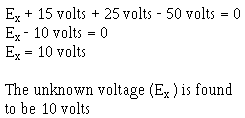Figure 3-23. - Determining unknown voltage in a series circuit.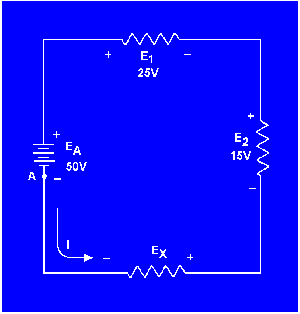Using the same idea as above, you can solve a problem in which the current is the unknown quantity.

Example: A circuit having a source voltage of 60 volts contains three resistors of 5 ohms, 10 ohms, and 15 ohms. Find the circuit current.

Solution: Draw and label the circuit (fig. 3-24). Establish a direction of current flow and assign polarities. Next, starting at any point - point A will be used in this example - write out the loop equation.

Figure 3-24. - Correct direction of assumed current.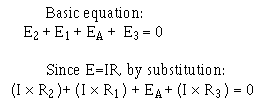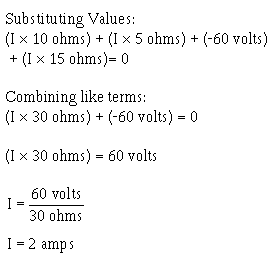Since the current obtained in the above calculations is a positive 2 amps, the assumed direction of current was correct. To show what happens if the incorrect direction of current is assumed, the problem will be solved as before, but with the opposite direction of current. The circuit is redrawn showing the new direction of current and new polarities in figure 3-25. Starting at point A the loop equation is: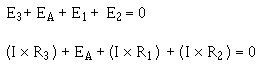Figure 3-25. - Incorrect direction of assumed current.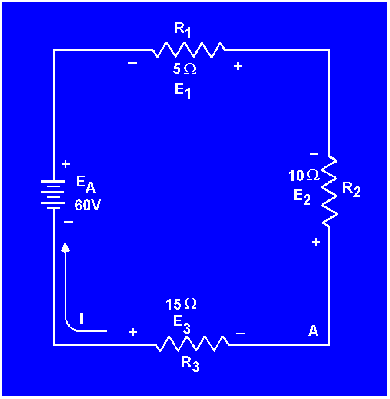Notice that the AMOUNT of current is the same as before. The polarity, however, is NEGATIVE. The negative polarity simply indicates the wrong direction of current was assumed. Should it be necessary to use this current in further calculations on the circuit using Kirchhoff's law, the negative polarity should be retained in the calculations.